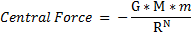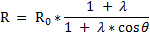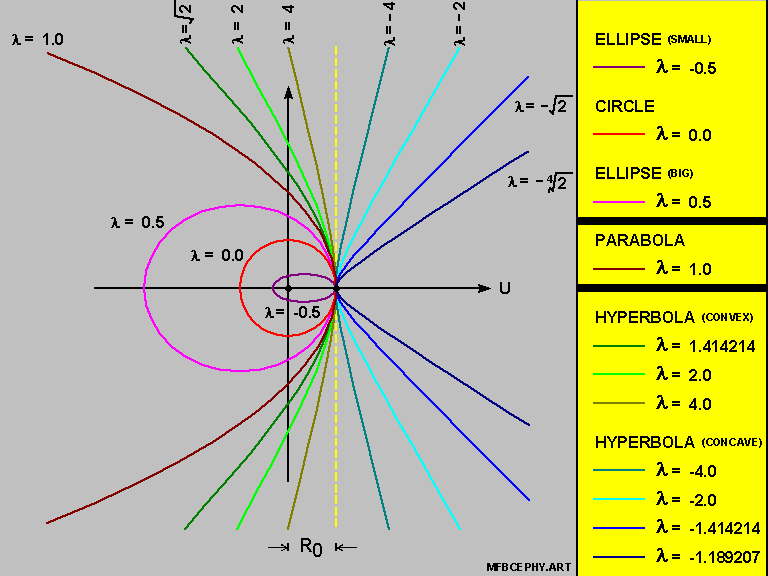Mathematic Functions Basics HomePage

# An Approach to Mathematic Functions Basics

### Summary :

• This paper was originally intended as an in-depth study of several Mathematic Functions ,

• in preparation of a full attack on the Riemann Zeta Function .

But we veered off-course and found something interesting :

• In Part VI of the papar , we looked at the Orbit of a Satellite moving under a Central Force ,

•And we threw around the idea that :

• The Circle-Ellipse-Parabola-Hyperbola combination

• is nothing more than a { Planar Cross-Section Cut } of 3-Dimensional Curves

• for the value of [ N ] being equal to [ 2 ] ;

and

• there are other { Planar Cross-Section Cuts } for [ N = 3 ] , [ N = 4 ] , etc. .

( see Key Finding 2 below for more details )

#### Key Finding One :

• The Circle , Ellipse , Parabola , Hyperbola combination can be covered via a single type of equation of the format :

•in Polar Co-ordinates .#### Key Finding Two :

• We looked at the orbit of a Satellite moving under a Central Force :

•We do observe that :

• the Circular Orbit is always a possibility for { all values of [ N ] greater than [ zero ] } ;

• and when the Mass-at-the-Center disappears , the Satellite shoots-off in a straight-line .

The Circular Orbit and the Straight-Line Orbit are therefore anchoring orbital-shapes for { all values of [ N ] greater than [ zero ] } .

As such ,

• this opens up the possibility of standardization and therefore a linkage system of orbital-shapes

• throughout the entire range for [ N ] .

#### Table of Content

Part I --- Introduction and Preliminaries Part II --- The Logarithmic Functions Section I Introduction Section II A Statement of Purpose for Part II Section III Deriving the Logarithmic Functions Section IV Defining Natural Logarithms Section V Flexibility inherent within the Logarithmic Class of Functions Section VI Evaluating Logarithms via an Infinite Series Section VII The Logarithms of Natural Numbers Section VIII A Statement of Purpose for Part III Section IX The Hyperbola in Cartesian Co-ordinates Section X The Geometric Definition of the Hyperbola Section XI Equation of the Hyperbola in [ U-V Axis Co-ordinates ] Section XII Equation of the Hyperbola in [ Polar Co-ordinates ] Section XIII The Hyperbola via [ Focal-Point based Co-ordinates ] - Part I Section XIV The Hyperbola via [ Focal-Point based Co-ordinates ] - Part II Section XV The Asymptotes for the Hyperbola - Part I Section XVI The Asymptotes for the Hyperbola - Part II Section XVII A Statement of Purpose for Part IV Section XVIII Geometric Definition of the Ellipse Section XIX Equation for the Ellipse - Part I Section XX Equation for the Ellipse - Part II Section XXI Equation for the Circle Section XXII Equation for the Parabola Section XXIII Linkage System for the Circle / Ellipse / Parabola / Hyperbola Section XXIV The Reasons for bringing-in the Orbit of a Satellite Section XXV A Statement of Purpose for Part VI Section XXVI Setting up the Polar Co-ordinates Reference Frame Section XXVII Setting up the Central Force influencing the Satellite Section XXVIII The Differential Equation involving [ R ] and [ Theta ] Section XXIX Setting up the Standardized Set of Initial Conditions Section XXX The Case of the Circular Orbit as the Base Scenerio Section XXXI The Case of the Central Force disappearing totally Section XXXII Solving for [ R ] in terms of [ Theta ] Section XXXIII Summarizing on the Orbit of a Satellite Section XXXIV Other Central Forces of a Similar Nature Section XXXV Re-doing the Differential Equation for [ N = 2 ] Section XXXVI Doing the Differential Equation for [ N = 3 ] Section XXXVII A Graphic Look at the Solutions for [ N = 3 ] Section XXXVIII Some Observations on the Orbits of the Satellite Section XXXIX A Proposal for Further Study of Orbital Shapes Section XL A Statement of Purpose for Part VII Section XLI Deriving the Tangent Addition Formula Section XLII The Tangent Addition Formula in Action Section XLIII Tangent Additions and the Pascal Triangle Section XLIV Algebraic Equations arising from { Tangent of [ Pi-over-4 ] = 1 } Section XLV A Statement of Purpose for Part VIII Section XLVI Deriving the Arctangent Function Section XLVII Using the Ratio of Areas to define an Angle Section XLVIII Some Graphics related to the Arctangent Function Section XLIX Concluding Remarks